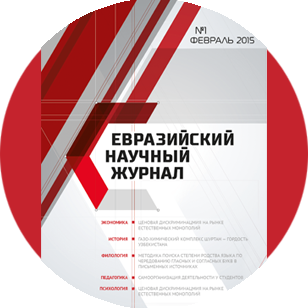Евразийский
научный
журнал

Срочная публикация научной статьи

+7 995 770 98 40
+7 995 202 54 42
info@journalpro.ru# METHODS OF TEACHING QUANTITIES IN ELEMENTARY MATHEMATICS LESSONSПоделитесь статьей с друзьями:
Автор(ы): Raximova Musharraf Komiljanovna
Рубрика: Педагогические науки
Журнал: «Евразийский Научный Журнал №2 2020»  (февраль, 2020)
Количество просмотров статьи: 1074
METHODS OF TEACHING QUANTITIES IN ELEMENTARY MATHEMATICS LESSONS

Raximova Musharraf Komiljanovna
Uychi district - teacher of secondary school № 1,
Namangan, Uzbekistan

In 2017-2021, the strategy of action on the five priority directions of the development of the Republic of Uzbekistan in the priority directions of the development of the social sphere was determined to improve the state policy of young people, to educate young people who are healthy, spiritually and mentally developed, think independently, are loyal to the Motherland, have a strict life outlook,

Quantities, just like numbers, are the basic concept of mathematics lessons in primary classes, and in children, subjects about quantity and related to reality and related to measurement are used for qualitative imagination.

In the initial classes, quantities such as length, body whiteness, volume, time, face of the figura are considered such concepts as value, quantity, price, speed, distance, productivity. Quantitative concept is one of the basic concepts studied in mathematics. Students should form a clear picture of these quantities, get acquainted with their units of measurement, learn how to measure quantities, express the results of measurements in different units, perform arithmetic operations on the basis of the named numbers. a snow-covered quantity is a generalized property of the real world in the environment. Exercise on measurementtiradi develop spatial imaginations. Armed with the necessary practical skills that are widely used in the life of students. The study of quantities is one of the selective feeding tools of Education. In elementary classes, the study of basic quantities is carried out in the most complete immortality with the study of arithmetic materials. Learning to measure is fed by learning to count. The new units of measurement are studied after the introduction of the corresponding units of counting. The number of nouns is denoted by the number of times the number is denoted by the number of times the number is written, read. Arithmetic actions are performed on numbers and on named numbers. Accuracy and adherence to batter in the performance of various calculations and conjunctions have an instructive acumen, in addition to educational acumen. The metered measurement and graphic work allows children to be brought up aesthetically, reducing their fatigue, increasing attention.

To formulate the outlines of quantities, practical methods and laboratory work are used. In the texturing and thorough formation of the outlines about the quantity, various tools of indication, that is, models of geometric figuresis, various drawing and measuring instruments are used.

It would be desirable to emphasize the general stages in the study of each quantity separately, even if he or she had an idea of the quantity and the methods of forming and measuring them had their own peculiarities. The teacher can rely on these in the process of studying each quantities and organize the activities of the students as follows.

The following methodological schemes can be used to teach quantities.

1. To identify the concepts of these quantities present in children (reference to the life experience of children).

2. Comparison of quantities with the same name (with the appearance of chameleons, comparison, comparison, measurement and other different methods).

3. Familiarization with the unit of measurement and measuring instruments of this quantity.

4. Formation of measurement skills in students.

5. Add and subtract quantities of the same name. (In the process of solving issues).

6. Introduce new units of measurement of quantities, convert quantities of one denominator into other units of measurement. Convert two units of measurement of the same name into one unit of measurement of two names, and vice versa.

7.Add and subtract the amount of two different names.

8.Multiply and divide the quantities by numbers.

We will dwell on the concepts that will die in the primary classes.

1. Length. The length of the incision. The initial reflection on the length as a feature of the predicate occurs in children as a schoolboy. When children come to school, they divide the subject by height, eni, height, the distance between them without errors, that is, they understand the meaning of longer, shorter, wider, narrower, longer, closer.

For example: blue tape is longer than red tape. The school is far from home. The apple tree is lower than the Poplar.

2. Mass (weight, weight).

In this section, students will get acquainted with the concept of mass (weight) and its unit of measurement — “kilograms”. It is necessary to approach the terms in the study of this topic with special care. The reason is that until recently they weighed the mass on a simple scale and called its indicators “weight”. But the mass and weight are not the same thing. Weight is the force that consists in multiplying the mass to acceleration. Therefore, when the children are asked the question "How Much Does this predicate weigh?"not saying, "how much is the mass of this subject?"it is permissible to ask. The question is put in such a form that it was introduced from the first class.

3. Volume.

With the concept of volume and its unit of measurement, the concept of liters, children get acquainted in the first class. In the 4th grade, simple views of the unit of measurement of volume are studied. Therefore, in the study of volume, there will be no such processes as the transition from one denominator to another denominator, addition and subtraction of the volume of two different denominators.